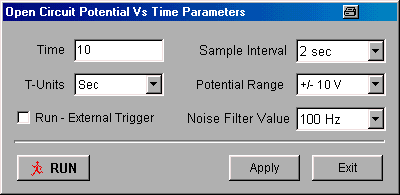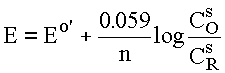# Open Circuit Potential Vs. Time

#### Introduction

The open circuit potential (also referred to as the equilibrium potential, the rest potential, or the corrosion potential) is the potential at which there is no current; that is, experiments based on the measurement of the open circuit potential are potentiometric experiments. Although such measurements are very simple, they have many important applications, which are briefly discussed below. The open circuit measurement technique is standard on the epsilon.

#### Setting Up an Open Circuit Measurement

Setting up an open circuit potential vs. time experiment is very simple. The important parameters that need to be entered by the user (in the appropriate Change Parameters dialog box (Fig1) from either the Experiment menu or the pop-up menu) are the length of the experiment (defined by Time in units of seconds or minutes (as specified by T-Units)) and the Sample Interval, which determines how often a data point is acquired. The largest number that can be entered for Time is 32,000. However, the maximum number of data points that can be collected in one experiment is 64000, so the maximum value for the Time of a given experiment is also determined by the Sample Interval. For example, if T-Units = sec, and Sample Interval = 0.5 sec, then the maximum value for Time = 32,000. Note that the Multi-Run option is not available for this technique. If the Run - External Trigger box is checked, the experiment is started from an external device using the Start In back-panel connection.Figure 1. Change Parameters dialog box for open circuit potential measurements.

In addition to this measurement, the open circuit potential can be monitored using Measure Open Circuit Potential in the Experiment menu. Furthermore, this potential can be measured before another experiment (e.g., cyclic voltammetry) and can be automatically used as the initial potential.

#### Applications

The basis of potentiometric concentration measurements (e.g., for potentiometric titrations) is the Nernst equation, which relates the concentration of electroactive species at the electrode surface (Cs) to the potential at that electrode (E); that is, for the reaction O + e- = Rwhere Eo' is the formal redox potential of the electron transfer reaction. The potential E is measured between two electrodes: the indicator electrode and the reference electrode (the auxiliary electrode is disconnected for potentiometric measurements on the epsilon). The indicator electrode is selected such that its potential is sensitive to the concentration of the analyte in solution (e.g., a glass membrane electrode for the measurement of pH), and the reference electrode (e.g., the saturated calomel or the silver/silver chloride electrode) provides a stable reference potential for the measurement of the potential of the indicator electrode.

Other important open circuit potential measurements are the open circuit potential of a battery, and the equilibrium (corrosion) potential of a corroding system